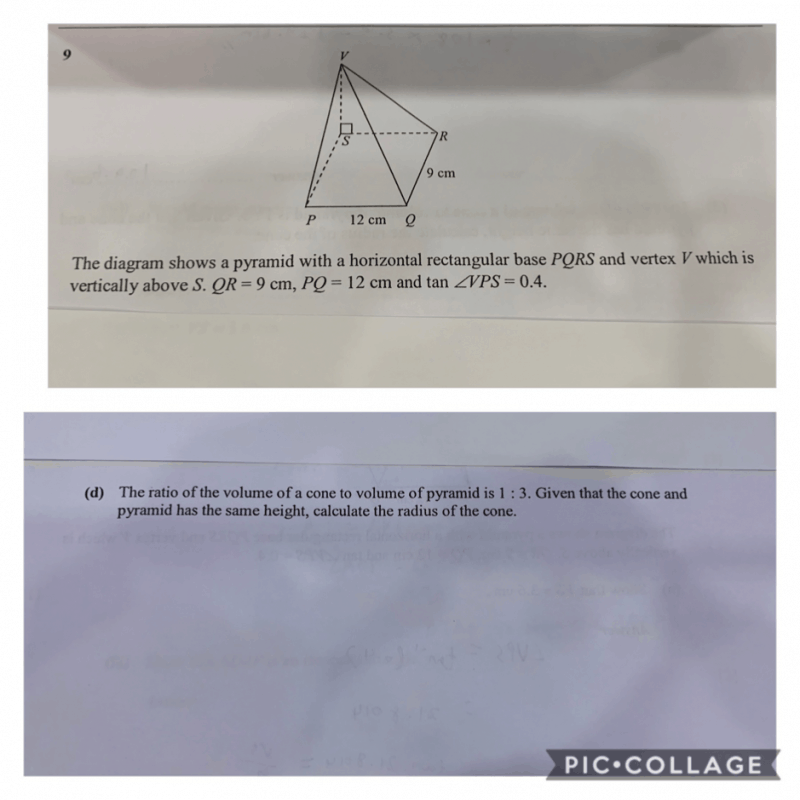# QuestionHow to do this S3 math. Thanks.

let the radius of cone be r.

volume of cone = pier^2h/3

volume of pyramid = abh/3 (a,b are length and breadth of rectangle)  = (12*9h)/3 = 28h

(pier^2h/3)/28h = 1/3

r^2/42 = 1/3

r = sqrt(14)